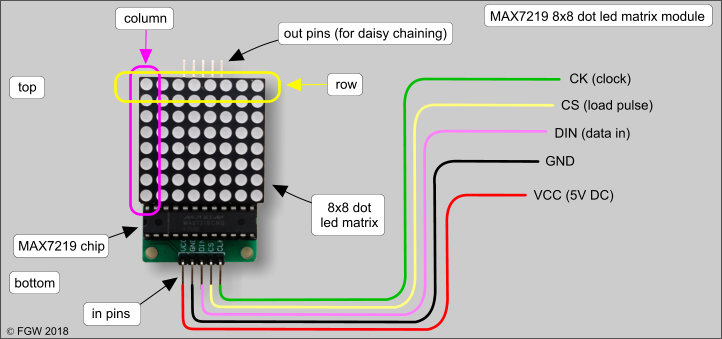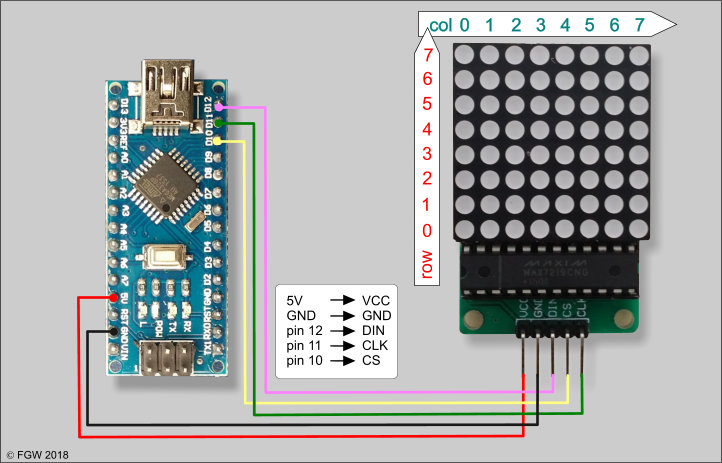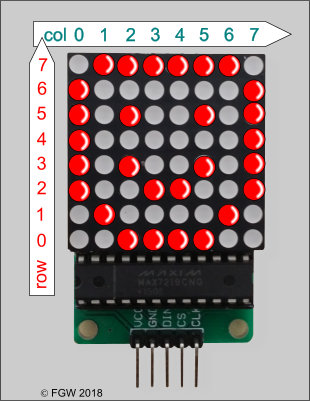# (7) Controlling a MAX7219 dot led matrix module with an Arduino Nano

by Floris Wouterlood – March 31, 2018

Summary
An 8×8 dot led matrix is a very interesting device because with proper instructions the individual leds or any group of leds can be programmed to fire. This characteristic, plus the feature that up to eight modules can be daisy chained, forms the basis for a nearly endless series of visual effects. Here we discuss the very basics of working with one MAX7219 dot led matrix module. A simple sketch is provided that creates a ‘smiley’ followed by a simulation of a bouncing ball.

Introduction
One of the first steps one does in the wonderful world of the Arduino is to light up leds. The most basic sketch here is ‘blink’, the series of instructions that controls sending power for a specified time to the Arduino’s built-in led. The first device I constructed with the help of an Arduino Uno, a breadboard, some leds, resistors, wires and the ‘blink’ example sketch was my first initial, the letter F. How proud I was to have achieved the magic of seeing my own initial blink in the dark!
Much more sophisticated than a single led is a led array. It is possible to construct a led array by soldering together a series of leds, but the problem is that every led needs one pin on the Arduino to control it. It is the number of available output pins of an Arduino which is the limiting factor. Because on an Arduino Uno and its little brother, the Nano in total 18 pins can be recruited to control leds, one can in theory control a maximum of 9×9 = 81 leds in an array with one Arduino Uno or its little brother. Yet it is possible to control many more leds via a special chip, called a shift register. For instance, the 74HC595 shift register chip is famous and very popular in the Arduino community. Yet I will be dealing in this paper with a MAX7219 shift register, mainly because the MAX7219 is a typical led driver included in a small, ready-to-go and very affordable module that includes a compact 8×8 dot led matrix (figure 1).Figure 1: MAX7219 dot led matrix module: a miniboard with a 8×8 dot led matrix, a MAX7219 shift register and, at the bottom, five connectivity pins: power (VCC; 5V DC), GND, clock (CLK), load pulse (CS) and data in (DIN). Via the five pins on the top edge a next module can be connected.

MAX7219 8×8 led dot matrix module: pins and connectivity with an Arduino Nano
Figure 2 shows the wiring of a MAX7219 module with an Arduino Nano. Apart from the 5V and GND wires there are only three output pins of the Arduino required. Very often, in fact so often that one can speak of a convention, is to connect CLK to pin 11 of the Nano, CS to pin 10 and DATA to pin 12. Other pins can be used as well. The selected pins used must be declared in the sketch anyway, if the sketch uses the ledControl.h library for compilation.Figure 2: Wiring of a MAX7219 module with an Arduino Nano (the wiring is the same for the Arduino Uno). Note that in most sketches available on the Internet the pin layout for CS,CLK and DIN are the same as shown here – a kind of convention. The pins assigned to CS, CLK and DIN need to be declared in the sketch (library: ledControl.h). Note that the Nano and the MAX7219 module are not in the same scale in this picture.

Electronics and supplies
1x MAX7219 8×8 dot led matrix module, 1x Arduino Nano, female-female Dupont wires.

Sketch
Below is the description and discussion of the complete ‘bare’ sketch. This sketch produces a ‘smiley’ followed by a sequence of leds that light up and switch off, simulating a bouncing ball. For programming an up- and running Arduino IDE on your computer is of course necessary. At the end of this paper a link is provided where to download this sketch. Note that the sketch reports the position of the firing led as output to Serial Monitor to the Arduino IDE on your computer.

Let’s go through the sketch

// singleMAX7219_bouncing_dot
//
// firing single leds in a 8×8 dot led matrix to simulate a bouncing dot
// random generator selects position dot on start
// March 26, 2018
// Floris Wouterlood
// public domain

#include “LedControl.h” // library that we need for this sketch and the MAX7219.

LedControl lc = LedControl (12,11,10,1);

• in the statement ‘LedControl lc’ the pins are declared that are being used: 12 is the DATA pin, 11 is the CLK pin, 10 is the CS pin and the ‘1’; stands for the fact there is only one module (up to 8 modules can be daisy chained with this library).
• now follows a series of variables that need to be declared. As the row- and column positions of the led that fires range between 0 and 7, a ‘byte’ type variable would be preferable; however, the library requires ‘int’ type variables which consume more dynamic memory. For the same token, ‘updown’ and ‘leftright’, which are the variables which determine whether a led in a position ‘up’ or ‘down’. ‘left’ or ‘right’ with respect to the current position will be fired, need do te declared as ‘int’.
• row_prev and col_prev together are the buffer in which the previous position of a firing led is stored. The sketch needs this data to switch that led off.

int row;
int row_prev;
int col;
int col_prev;
int ball_timer = 100; // time in between firing leds – ‘speed’ variable
long randNumber; // variable for the random generator
long updown=1; // directs movement: +1 is one row up up and -1 is one row down
long leftright=1; // directs movement: +1 is one col right and -1 is one col left

Next a character, named smiley[], is defined that fills the complete 8×8 dot led matrix with zeros and ones: the smiley character.This smiley is composed as a kind of 8×8 bitmap: a 0 is a ‘silent led while a 1 is a firing led. The firing leds together form the ‘smiley’. Note that if you compare this display with the byte smiley[] array you will see that the smiley as you see it on the MAX7219 unit, is turned 90 degrees with the horizontal compared with the byte smiley [] matrix. The reason for this is that the LedControl library uses a function ‘setRow’ to build up the smiley while a function ‘setColumn’ would have been better. However, the library does not provide a ‘setColumn’ function.

byte smiley[] = // fill array for the smiley
{
B00111110,
B01000001,
B10010101,
B10100001,
B10100001,
B10010101,
B01000001,
B00111110
};

After declaring all variables the sketch continues with the portion that runs only once.

void setup() {

Serial.begin (9600);
// the MAX72XX is in power-saving mode on startup,
// we have to do a wakeup call

lc.shutdown (0,false);

// set the brightness to a medium value
lc.setIntensity (0,0); // 0 = low; 8 = high

• in case there are more than one MAX7219 daisy chained, the first of the two digits indicates of which module the intensity is to be set.

// show smiley – to demonstrate that the 8×8 array works
smile ();
delay (1000);

// clear the display // goodbye smiley!
lc.clearDisplay(0); // the ‘0’ stands for the first module in a daisy chain

• also here the index indicates which unit is involved (0-7)

// initial position
col = random (1, 7); // sets a col position between 1 and 7
row = random (1, 7); // sets a row position between 1 and 7

// display start col and row numbers on serial monitor
Serial.println (“start condition” );
Serial.print (“row: “);
Serial.println (row);
Serial.print (“col: “);
Serial.println (col);
Serial.println (“=======”);
Serial.println ();
}

// here is the series of instructions for the continuously running part of the sketch

void loop() {

col_prev= col;            // log position of ball
row_prev = row;        // log position of ball
row = row+updown; // calculate new position of ball
col = col+leftright;    // calculate new position of ball

checkVectors ();

lc.setLed(0, col_prev, row_prev, false); // erase previous ball position
lc.setLed(0, col, row, true); // light up new ball position
delay(ball_timer);

debug(); // run the debug module

}
// ============ SUBROUTINES ===================================

void smile() // here we build up the smiley, row after row
{
for (int i = 0; i < 8; i++)
{
lc.setRow(0,i,smiley[i]);
}
}

• the subroutine checkVectors detects when a ball tries to jump over one of the four sides of the matrix, let it reverse course and positions the bal 1 position back in the respective column or row in the matrix

void checkVectors () {

if ((row>7) && (updown=1)){
updown=-1;
row=6;
}

if ((row<0) && (updown=-1)){
updown=1;
row=1;
}

if ((col>7) && (leftright=1)){
leftright=-1;
col=6;
}

if ((col<0) && (leftright=-1)){
leftright=1;
col=1;
}
}

void debug (){

Serial.print (“col: ” );
Serial.println (col);
Serial.print (“row: ” );
Serial.println (row);
}

Sketch